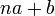# Composition length

(diff) ← Older revision | Latest revision (diff) | Newer revision → (diff)

This article defines an arithmetic function on a restricted class of groups, namely: group of finite composition lengths

## Definition

### Symbol-free definition

The composition length of a group is defined as the length of any composition series of the group. The composition length is well-defined only for a group of finite composition length.

By the Jordan-Holder theorem, the composition length is well-defined, because all composition series have the same length and in fact the same collection of composition factors.

### Definition with symbols

Suppose$G$ is a group with a composition series:$\{ e \} = N_0 \le N_1 \le N_2 \le \dots \le N_r = G$.

Then, the composition length of$G$ is defined to be equal to$r$.

## Facts

### Composition lengths related to some group properties

Group property or condition Meaning What we can say about the composition length
trivial group group of order one, has only the identity element it is 0. In fact, the trivial group is the only group of composition length 0.
simple group no proper nontrivial normal subgroup it is 1. In fact, a group is simple if and only if it has composition length 1.
finite group the underlying set is finite The composition length is finite, and is bounded by the sum of the exponents on all prime divisors in a prime factorization.
finite solvable group finite and solvable, so in particular all the composition factors are groups of prime order. The composition length equals the sum of the exponents on all prime divisors in a prime factorization. Specifically, if the order is$p_1^{k_1}p_2^{k_2} \dots p_r^{k_r}$ with$p_1,p_2,\dots,p_r$ distinct primes, then the composition length is$k_1 + k_2 + \dots + k_r$.
In fact, the composition length is$k_1 + k_2 + \dots + k_r$ if and only if the group is solvable.
group of prime power order order is a prime power, i.e., of the form$p^k$,$p$ prime. composition length is$k$, i.e., the prime-base logarithm of order.

### Relation with product notions

Product notion Information for factors or inputs to the product Result for product notion Proof
external direct product$G_1$ has composition length$a_1$,$G_2$ has composition length$a_2$$G_1 \times G_2$ has composition length$a_1 + a_2$ composition length of direct product is sum of composition lengths
external semidirect product$N$ has composition length$a$, and$H$ has composition length$b$, with$\rho:H \to \operatorname{Aut}(N)$ an action$N \rtimes H$ has composition length$a + b$ composition length of semidirect product is sum of composition lengths
group extension$N$ has composition length$a$,$G/N$ has composition length$b$$G$ has composition length$a + b$ composition length of extension group is sum of composition lengths
wreath product$A$ has composition length$a$,$B$ has composition length on a set of size$b$ and its permutation action is on a set of size$n$$A \wr B$ has composition length$na + b$